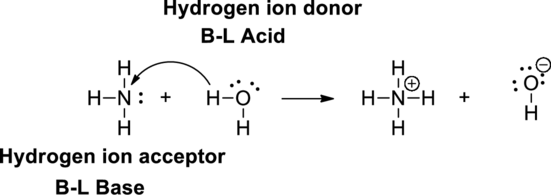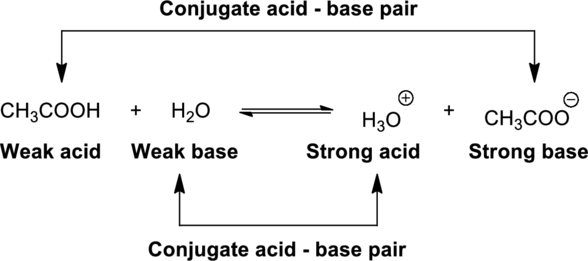# (a) Which is the stronger Bronsted acid, HCO 3 − or NH 4 + ? Which has the stronger conjugate base? (b) Is a reaction between HCO 3 - ions and NH 3 product- or reactant-favored at equilibrium? HCO 3 − (aq) + NH 3 (aq) ⇄ CO 3 2− (aq) + NH 4 + (aq) (c) You mix solutions of sodium hydrogen phosphate and ammonia. The net ionic equation for a possible reaction is HPO 4 2‒ (aq) + NH 3 (aq) ⇄ PO 4 3‒ (aq) + NH 4 + (aq) Does the equilibrium lie to the left or to the right for this reaction?### Chemistry & Chemical Reactivity

9th Edition
John C. Kotz + 3 others
Publisher: Cengage Learning
ISBN: 9781133949640

#### Solutions

Chapter
Section### Chemistry & Chemical Reactivity

9th Edition
John C. Kotz + 3 others
Publisher: Cengage Learning
ISBN: 9781133949640
Chapter 16.5, Problem 1CYU
Textbook Problem
202 views

## (a) Which is the stronger Bronsted acid, HCO3− or NH4+? Which has the stronger conjugate base? (b) Is a reaction between HCO3- ions and NH3 product- or reactant-favored at equilibrium? HCO3−(aq) + NH3(aq) ⇄ CO32−(aq) + NH4+(aq)(c) You mix solutions of sodium hydrogen phosphate and ammonia. The net ionic equation for a possible reaction isHPO42‒(aq) + NH3(aq) ⇄ PO43‒(aq) + NH4+(aq)Does the equilibrium lie to the left or to the right for this reaction?

(a)

Interpretation Introduction

Interpretation:

The stronger Bronsted acid between HCO3 and NH4+ and the stronger conjugate base has to be stated.

Concept Introduction:

Bronsted –Lowry definitions:

A Bronsted–Lowry acid is a proton donor, it donates a hydrogen ion, H+

A Bronsted-Lowry base is a proton acceptor, it accepts a hydrogen ion H+.Bronsted–Lowry conjugate acid-Base pairs:

When an acid is dissolved in water, the acid (HA) donates a proton to water to form a new acid (conjugate acid) and a new base (conjugate base).An equilibrium constant (K) is the ratio of the concentration of products and reactants raised to appropriate stoichiometric coefficient at equilibrium.

The reaction of any acid HA with water is written as,

HA(aq)+H2O(l)H3O+(aq)+A(aq)

The relative strength of an acid and base in water can be also expressed quantitatively with an equilibrium constant as follows:

Ka=[H3O+][A][HA]

An equilibrium constant (K) with subscript a indicates that it is an equilibrium constant of an acid in water.

The reaction of any base B with water is written as,

B(aq)+H2O(l)BH(aq)+OH(aq)

The relative strength of an acid and base in water can be also expressed quantitatively with an equilibrium constant as follows:

Kb=[BH][OH][B]

An equilibrium constant (K) with subscript b indicates that it is an equilibrium constant of the base in water.

### Explanation of Solution

The dissociation constant (Ka) for HCO3 is 4.8×1011.

The dissociation constant (Ka) for NH4+ is 5.6×1010.

The reaction of HCO3 with water is written as,

HCO3(aq)+H2O(l)H3O+(aq)+CO32(aq)

The dissociation constant for HCO3 ion is expressed as,

Ka=[H3O+][CO32][HCO3]

A smaller value of Ka=4.8×1011 indicates that the ionization product is weakly favored because the product [H3O+][CO32] is less than the equilibrium concentration of the weak acid [HCO3]

(b)

Interpretation Introduction

Interpretation:

The reaction between HCO3 and NH3 at equilibrium is a product favored or a reactant favored has to be stated.

Concept Introduction:

An equilibrium constant (K) is the ratio of the concentration of products and reactants raised to appropriate stoichiometric coefficient at equilibrium.

The reaction of any acid HA with water is written as,

HA(aq)+H2O(l)H3O+(aq)+A(aq)

The relative strength of an acid and base in water can be also expressed quantitatively with an equilibrium constant as follows:

Ka=[H3O+][A][HA]

An equilibrium constant (K) with subscript a indicates that it is an equilibrium constant of an acid in water.

The reaction of any base B with water is written as,

B(aq)+H2O(l)BH(aq)+OH(aq)

The relative strength of an acid and base in water can be also expressed quantitatively with an equilibrium constant as follows:

Kb=[BH][OH][B]

An equilibrium constant (K) with subscript b indicates that it is an equilibrium constant of the base in water.

(c)

Interpretation Introduction

Interpretation:

The reaction between HPO42 and NH3 at equilibrium is a product favored or a reactant favored reaction has to be stated.

Concept Introduction:

An equilibrium constant (K) is the ratio of the concentration of products and reactants raised to appropriate stoichiometric coefficient at equilibrium.

The reaction of any acid HA with water is written as,

HA(aq)+H2O(l)H3O+(aq)+A(aq)

The relative strength of an acid and base in water can be also expressed quantitatively with an equilibrium constant as follows:

Ka=[H3O+][A][HA]

An equilibrium constant (K) with subscript a indicates that it is an equilibrium constant of an acid in water.

The reaction of any base B with water is written as,

B(aq)+H2O(l)BH(aq)+OH(aq)

The relative strength of an acid and base in water can be also expressed quantitatively with an equilibrium constant as follows:

Kb=[BH][OH][B]

An equilibrium constant (K) with subscript b indicates that it is an equilibrium constant of the base in water.

### Still sussing out bartleby?

Check out a sample textbook solution.

See a sample solution

#### The Solution to Your Study Problems

Bartleby provides explanations to thousands of textbook problems written by our experts, many with advanced degrees!

Get Started

Find more solutions based on key concepts
When energy from food is in short supply the body dismantles its glycogen and releases triglycerides for energy...

Nutrition: Concepts and Controversies - Standalone book (MindTap Course List)

Speed is a(n) ___ quantity. (2.2)

An Introduction to Physical Science

How is heat different from temperature?

Oceanography: An Invitation To Marine Science, Loose-leaf Versin

The intensity of a sound wave at a fixed distance from a speaker vibrating at 1.00 kHz is 0.600 W/m2. (a) Deter...

Physics for Scientists and Engineers, Technology Update (No access codes included)